cancel
Showing results for
Show  only  | Search instead for
Did you mean:
Choose Language Hide Translation Bar

## Wilcoxon signed rank test : Test statistic S

Hello everyone,

I apologize in advance for what must seem like a very obvious question.

When you do a pair analysis of variable in JMP, you can ask the software to perform a Wilcoxon signed rank test which then gives me as a result a test statistics named S.

Question is : What is S?

It does not match the sum of all the ranks because it can be a negative

It does not match the sum of the signed ranks or even the half-sum of the signed ranks (and it would be named W not S)

Can someone please share some light on this issue since the JMP documentation only states how to calculate W but doesn't say a word about S ?

Another question : How do i switch from a one-tail test (which is the one performed) to a two tailed test.

I've included the sample data i use. The sum of the positive ranks is 19 and the sum of the negative ranks is 134.

W should be 62.5. S is 68. What am i missing ?

Best regards,

1 ACCEPTED SOLUTION

Accepted Solutionsmichael_jmp
Staff

## Re: Wilcoxon signed rank test : Test statistic S

I should point out that while JMP uses the Pratt method to address zero differences, SAS does not use the Pratt method.

There was some confusion from the documentation, since it uses W instead of S. I have worked on updating the documentation for JMP 15 to use S, as well as to correct some of the formulas there.

The Pratt method adjustment is the d0(d0+1) term in the second definition of S.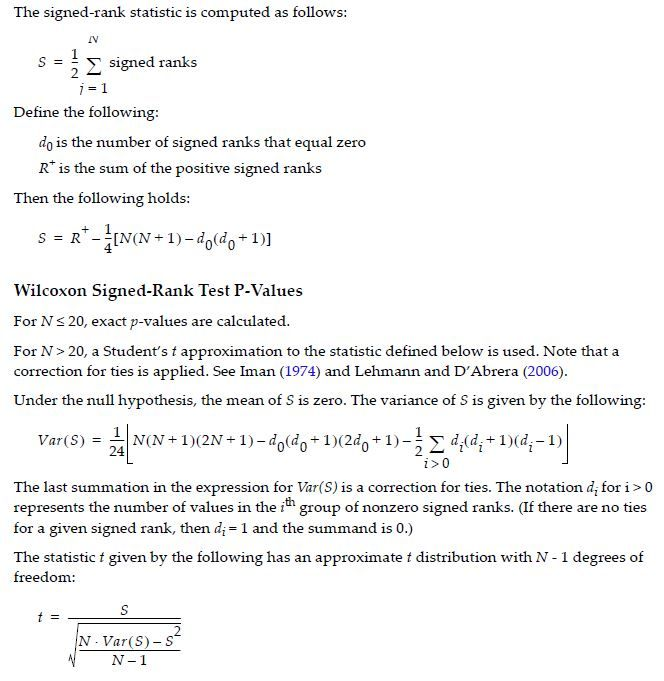Hope this helps.

Michael

Michael Crotty
Sr Statistical Writer
JMP Development
11 REPLIES 11

## Re: Wilcoxon signed rank test : Test statistic S

Do you have any 0 differences? You could look up the references that are cited in the JMP Help:

Wilcoxon Signed Rank
Shows or hides the Wilcoxon signed rank test. The Wilcoxon signed rank test is applied to the paired differences. It is a nonparametric test that compares the sizes of the positive differences to the sizes of the negative differences. The test uses the Pratt method to address zero differences. The test also assumes that the distribution of differences is symmetric. For details, see Distributions in the Basic Analysis book. See also Lehman (2006), Conover (1999, p. 350), and Cureton (1967).

I see this result in my example: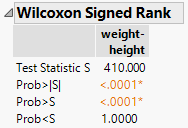The two-tailed and both one-tailed tests are available.

## Re: Wilcoxon signed rank test : Test statistic S

Hello @Mark_Bailey ,

Thank you for your answer, i now understand that both one and two-tailed tests are performed and both results are given.

Now back to "S" :

I did indeed checked the JMP help and here are the two formulas i can find: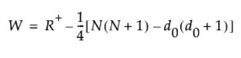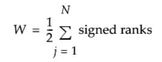I have one 0 difference which means d0 = 1 and N = 18.
The sum of positive ranks gives me 139 and the sum of negative ranks being 14.

The formula on the right returns (14-139)/2 = -62.5 , the formula on the left returns 14-(18*17-2)/4=-62

None of them gives me -68, i believe this is because these are the formulas for W not S, and S isn't mention in the JMP help section of the Wilcoxon signed rank test.

It's still very unclear to me why that S statistics appears instead of W and why it's different from the Wilcoxon test statistic.

Is there any way to display the details of the calculations steps (rank numbering, ...), maybe it could shed some light on this issue ?

Best regards,

## Re: Wilcoxon signed rank test : Test statistic S

This information comes from the Base SAS documentation for PROC UNIVARIATE. All the symbols were unfortunately lost in the copy and paste operation but you can see the full explanation with the link provided.

I believe that the same computation is used by JMP but if you still find disagreement between your result and JMP, contact JMP Technical Support.

#### Wilcoxon Signed Rank Test

The signed rank statistic S is computed as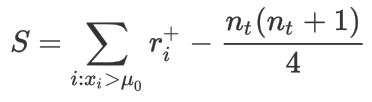where is the rank of after discarding values of , and is the number of values not equal to . Average ranks are used for tied values.

If , the significance of S is computed from the exact distribution of S, where the distribution is a convolution of scaled binomial distributions. When , the significance of S is computed by treating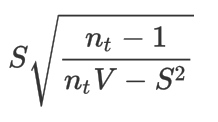as a Student’s t variate with degrees of freedom. V is computed as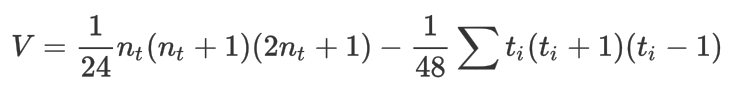where the sum is over groups tied in absolute value and where is the number of values in the ith group (Iman 1974; Conover 1980). The null hypothesis tested is that the mean (or median) is , assuming that the distribution is symmetric. Refer to Lehmann and D’Abrera (1975).

## Re: Wilcoxon signed rank test : Test statistic S

Hello @Mark_Bailey ,

Thank you again for your help in this matter.

I believe you actually found the formula used by JMP to calculate S.

However when i apply it with R+ = 139 and N = 17 i still do not find the infamous "-68" that JMP gives me.

I have tried all day to figure out what my error could be and checked my results against every online Wilcoxon calculator.

I even tried to with different values of N but still no luck...

I will contact JMP support and hope for the best.

As soon as i have an answer from them i will update this thread

best regards,

## Re: Wilcoxon signed rank test : Test statistic S

I just wanted to point out something which i believe could lead to an explanation :

For every sample where there is no tied value (i.e. equal value before and after), then S=W and the formulas given above are correct and it is the result given by JMP.
When i delete the tied value from my sample and submit it to JMP for a Wilcoxon signed rank test, the statistics result is the correct one : S=-62.5

The issue only occurs when there is a tied value in the sample.

For anyone trying to reproduce the problem, using the roadtest sample in the matched pairs tutorial. The Wilcoxon result is correct but once you add a tied pair (by setting Kira's after result to 6 for example) then the S result is not equal to W and deviates from what is expected from the formulas.

## Re: Wilcoxon signed rank test : Test statistic S

As I pointed out in my first reply, the SAS and JMP test uses the Pratt method to address zero differences.

## Re: Wilcoxon signed rank test : Test statistic S

Hello @Mark_Bailey ,

My mistake, you were right to remind me.

I had some troubles finding out about the Pratt Method and what it does exactly but after some trial and error i was able to find W = -68 as intended.

Thank you again for your help.

Case solved

Best regards,

## Re: Wilcoxon signed rank test : Test statistic S

Could you help me with this? I am having you same issue and I just cant figure this out. My Sum of positive ranks is 1527, my N = 76, i have 10 0's.michael_jmp
Staff

## Re: Wilcoxon signed rank test : Test statistic S

I should point out that while JMP uses the Pratt method to address zero differences, SAS does not use the Pratt method.

There was some confusion from the documentation, since it uses W instead of S. I have worked on updating the documentation for JMP 15 to use S, as well as to correct some of the formulas there.

The Pratt method adjustment is the d0(d0+1) term in the second definition of S.Hope this helps.

Michael

Michael Crotty
Sr Statistical Writer
JMP Development